Question

# a. Using the Ksp value for Cu(OH)2 (1.6 x 10-19) and the overall formation constant for...

a. Using the Ksp value for Cu(OH)2 (1.6 x 10-19) and the overall formation constant for Cu(NH3)42+ (1.0 x 1013), calculate the value for the equilibrium constant for the following reaction:

Cu(OH)2 (s) + 4NH3 (aq) ⇌ Cu(NH3)42+(aq) + 2OH -(aq)

b. Use the value of the equilibrium constant you calculated in part a to calculate the solubility (in mol/L) of Cu(OH)2 in 5.0 M NH3. In 5.0 M NH3 the concentration of OH - is 0.0095 M.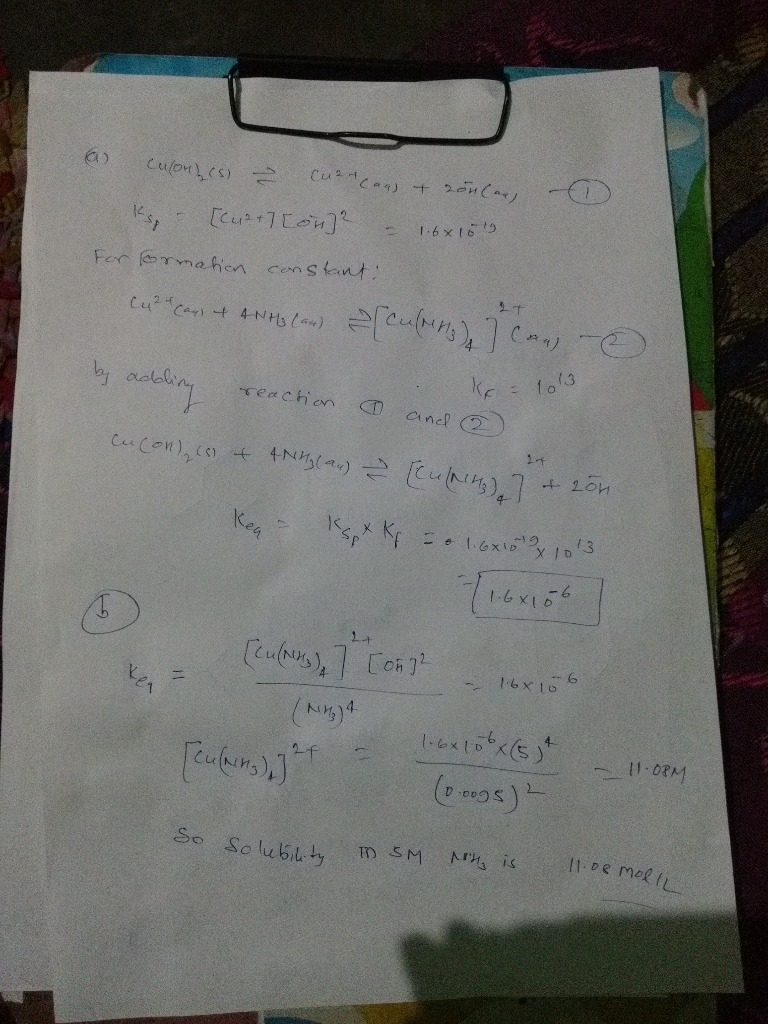#### Earn Coins

Coins can be redeemed for fabulous gifts.

Similar Homework Help Questions
• ### please I need help with these 2 questions CHEM 112 Second Chapter 16 Worksheet 1. Calculate...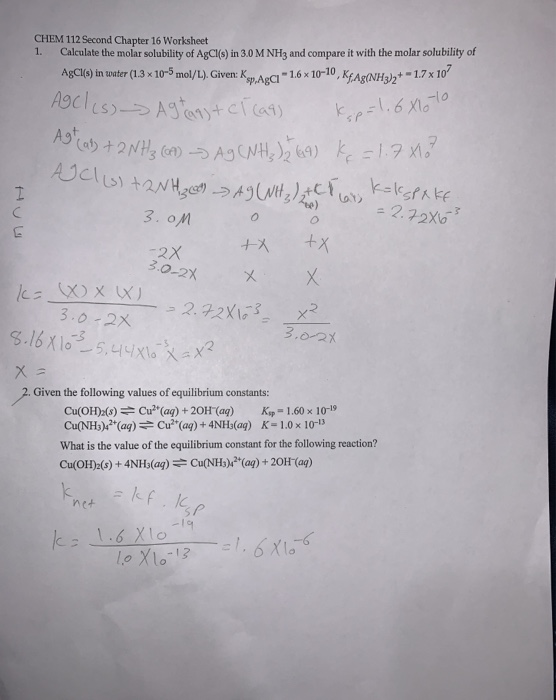please I need help with these 2 questions CHEM 112 Second Chapter 16 Worksheet 1. Calculate the molar solubility of AgCl(s) in 3.0 M NH3 and compare it with the molar solubility of AgCl(s) in water (1.3 x 10-5 mol/L). Given: Kg.Aga - 1.6 x 10-10, KfAg(NH3)2+ - 1.7x10' AgCl (s) > Agtags + Cl cas kap=1.6 X10 Ago (at) + 2NH3 (61) - Ag CN Hz ) 169) ķ=1.7x10² Ucl(s) + 2NH2 con A9 (WHz / 2+1 Lars Katspake...

• ### If Cu(OH)2 has Ksp = 1.6 x 10−19, what is the molar solubility of Cu(OH)2? a)...

If Cu(OH)2 has Ksp = 1.6 x 10−19, what is the molar solubility of Cu(OH)2? a) 5.1 × 10−10 M b) 6.4 × 10−7 M c) 2.7 × 10−11 M d) 1.7 × 10−10 M e) 3.4 × 10−7 M

• ### Question 2 If Cu(OH)2 has Ksp = 1.6 x 10-19, what is the molar solubility of...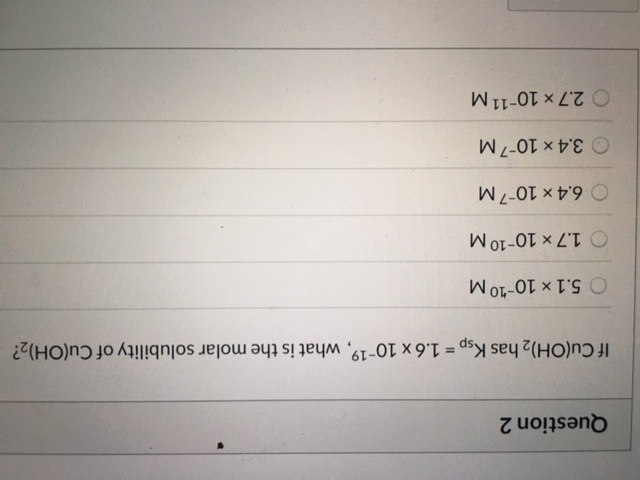Question 2 If Cu(OH)2 has Ksp = 1.6 x 10-19, what is the molar solubility of Cu(OH)2? 5.1 x 10-10 M. 1.7 x 10-10 M. 6.4 x 10-7M 3.4 x 10-7M 2.7 x 10-11 M.

• ### Given that the Ksp of CdCO3 is 1 x 10-12 and the formation constant of Cd(NH3)42+...

Given that the Ksp of CdCO3 is 1 x 10-12 and the formation constant of Cd(NH3)42+ is 1.0 x 107, what does the ammonia concentration of a solution need to be in order for the solubility of CdCO3 to be 0.1 M?

• ### a) The solubility product, Ksp, of Cd3(PO4)2 is 2.5 x 10-33. What is the solubility (in...

a) The solubility product, Ksp, of Cd3(PO4)2 is 2.5 x 10-33. What is the solubility (in g/L) of Cd3(PO4)2 in pure water? b) The solubility product of Cu(OH)2 is 4.8 x 10-20. Calculate the value of pCu2+, or -log[Cu2+], in an aqueous solution of NaOH which has a pH of 12.38 and is saturated in Cu(OH)2. c) The equilibrium constant for the formation of Cu(CN)42- is 2.0 x 1030. Calculate the value of pCu2+, or -log[Cu2+], if we were to...

• ### "Hydroxyapatite, Cao(PO)(OH),, has a solubility constant of Ksp = 2.34 x 10-59, and dissociates according to Ca,(PO...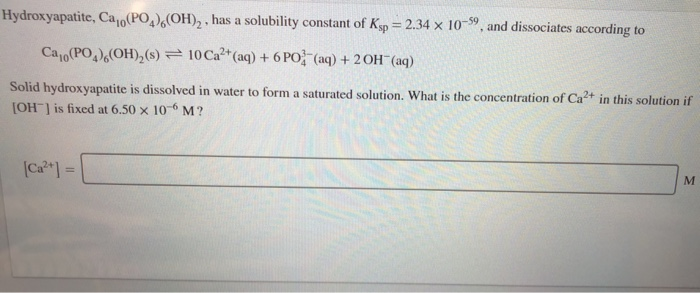"Hydroxyapatite, Cao(PO)(OH),, has a solubility constant of Ksp = 2.34 x 10-59, and dissociates according to Ca,(PO)(OH),(s) = 10 Ca2+ (aq) + 6 PO (aq) + 2OH(aq) Solid hydroxyapatite is dissolved in water to form a saturated solution. What is the concentration of Ca2+ in this solution if [OH-] is fixed at 6.50 x 10-M?

• ### 13. Calculate the value for the equilibrium constant, Ksp for the following reaction. Ca(OH)2 (s) -...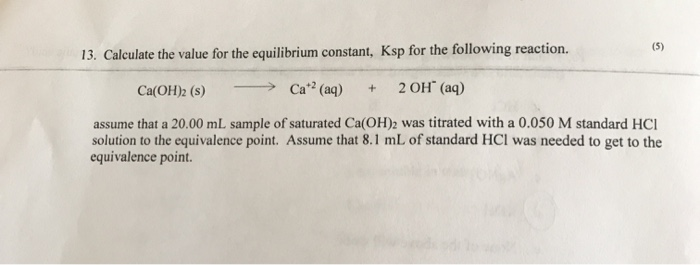13. Calculate the value for the equilibrium constant, Ksp for the following reaction. Ca(OH)2 (s) - > Cat? (aq) + 2OH(aq) assume that a 20.00 mL sample of saturated Ca(OH)2 was titrated with a 0.050 M standard HCI solution to the equivalence point. Assume that 8.1 mL of standard HCl was needed to get to the equivalence point

• ### Hydroxyapatite, Ca10(PO4)6(OH)2 , has a solubility constant of Ksp = 2.34×10−59 , and dissociates according to...

Hydroxyapatite, Ca10(PO4)6(OH)2 , has a solubility constant of Ksp = 2.34×10−59 , and dissociates according to Ca10(PO4)6(OH)2(s)↽−−⇀10Ca2+(aq)+6PO3−4(aq)+2OH−(aq) Solid hydroxyapatite is dissolved in water to form a saturated solution. What is the concentration of Ca2+ in this solution if [OH−] is fixed at 1.40×10−6 M ?

• ### Hydroxyapatite, Ca10(PO4)6(OH)2 , has a solubility constant of Ksp = 2.34×10−59 , and dissociates according to...

Hydroxyapatite, Ca10(PO4)6(OH)2 , has a solubility constant of Ksp = 2.34×10−59 , and dissociates according to Ca10(PO4)6(OH)2(s)↽−−⇀10Ca2+(aq)+6PO3−4(aq)+2OH−(aq) Solid hydroxyapatite is dissolved in water to form a saturated solution. What is the concentration of Ca2+ in this solution if [OH−] is fixed at 6.20×10−6 M ? [Ca2+]=

• ### (a) The solubility product, Ksp, for Sn (OH) 4 (s) is 1.0 x 10-57. What is...

(a) The solubility product, Ksp, for Sn (OH) 4 (s) is 1.0 x 10-57. What is its solubility (in g / L) in pure water? (b) For the reaction 2 A (aq) ⇋ B (aq) + C (aq), the value of ΔGo is 3.99 kJ at 25.0oC. If the initial concentrations A (aq), B (aq), and C (aq) are 0.416 M, 0.336 M, and 0.275 M respectively, what will be the concentration of A (aq) when we reach equilibrium at...